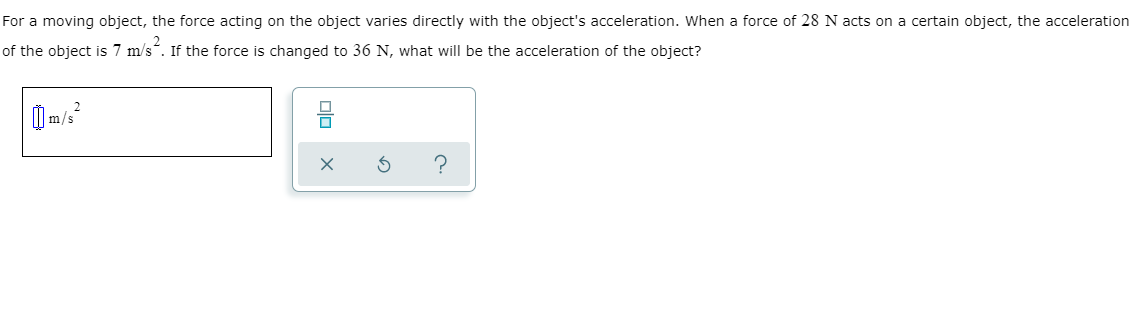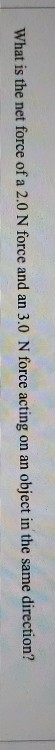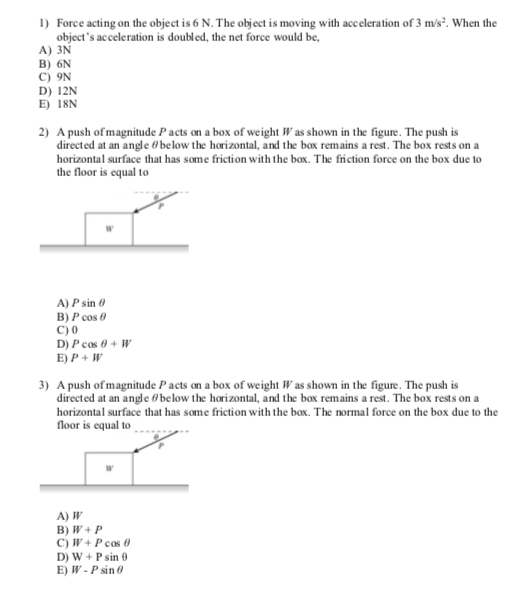Question

# What is the net force acting on an object moving at 100 mph due north? None...

What is the net force acting on an object moving at 100 mph due north?

None of the above

10 N south

10 N north

Net Force equals zero

Correct Ans:

Net force equal to zero

From Newtons law of motion, if an object is moving with a constant velocity(like 100 mph) in the given case, the net force is zero, as the acceleration is zero.

#### Earn Coins

Coins can be redeemed for fabulous gifts.

Similar Homework Help Questions
• ### For a moving object, the force acting on the object varies directly with the object's acceleration....For a moving object, the force acting on the object varies directly with the object's acceleration. When a force of 28 N acts on a certain object, the acceleration of the object is 7 m/s . If the force is changed to 36 N, what will be the acceleration of the object? ola x X 6 ?

• ### Three forces act on a moving object. One force has a magnitude of 78.6 N and...

Three forces act on a moving object. One force has a magnitude of 78.6 N and is directed due north. Another has a magnitude of 55.3 N and is directed due west. What must be (a) the magnitude and (b) the direction of the third force, such that the object continues to move with a constant velocity? Express your answer as a positive angle south of east.

• ### A plane is moving due north, directly towards its destination. Its airspeed is 210 mph. A...

A plane is moving due north, directly towards its destination. Its airspeed is 210 mph. A constant breeze is blowing from west to east at 20.0 mph. At what rate is the plane moving north?

• ### Object A is moving due east, while object B is moving due north. They collide and...

Object A is moving due east, while object B is moving due north. They collide and stick together in a completely inelastic collision. Momentum is conserved. Object A has a mass of mA = 18.0 kg and an initial velocity of v0A = 8.00 m/s, due east. Object B, however, has a mass of mB = 30.0 kg and an initial velocity of v0B = 5.00 m/s, due north. Find the magnitude of the final velocity of the two-object system...

• ### Object A is moving due east, while object B is moving due north. They collide and...

Object A is moving due east, while object B is moving due north. They collide and stick together in a completely inelastic collision. Momentum is conserved. Object A has a mass of mA = 16.8 kg and an initial velocity of = 7.37 m/s, due east. Object B, however, has a mass of mB = 29.0 kg and an initial velocity of = 5.03 m/s, due north. Find the (a) magnitude and (b) direction of the total momentum of the...

• ### Three forces act on a moving object. One force has a magnitude of 87.0 N and...

Three forces act on a moving object. One force has a magnitude of 87.0 N and is directed due north. Another has a magnitude of 44.6 N and is directed due west. What must be (a) the magnitude and (b) the direction of the third force, such that the object continues to move with a constant velocity? Express your answer as a positive angle south of east. (a) Number Units (b) Number Units

• ### a net force 28N is acting on a falling object. It experiences an air resistance of...

a net force 28N is acting on a falling object. It experiences an air resistance of 8.5 N. Accekeration due to gravity is 9.81 m/s2 what is the mass

• ### what is the net force of a 2.0 N force and an 3.0 N force acting...what is the net force of a 2.0 N force and an 3.0 N force acting on an object in the same direction? What is the net force of a 2.0 N force and an 3.0 N force acting on an object in the same direction?

• ### 1) Force acting on the object is 6 N. The object is moving with acceleration of...1) Force acting on the object is 6 N. The object is moving with acceleration of 3 m/s. When the object's acceleration is doubled, the net force would be, A) 3N B) 6N C) 9N D) 12N E) I8N 2) A push of magnitude P acts on a box of weight Was shown in the figure. The push is directed at an angle 0 below the horizontal, and the box remains a rest. The box rests on a horizontal surface...

• ### a 20.0-newton force due north and a 20.0-newton force due east act concurrently on an object, as...

a 20.0-newton force due north and a 20.0-newton force due east act concurrently on an object, as shown in the diagram below. The additional force necessary to bringthe object into a state of equilibrium is which of the following?20.0 N, southwest20.0 N, northeast28 N, northeast28 N, southwest